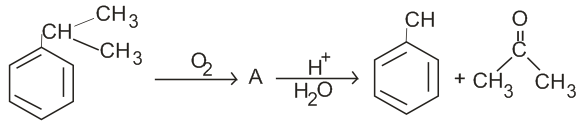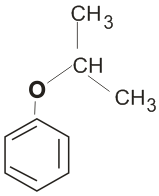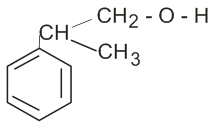## Filters

Sort by :
Q

A particle moving with velocity  is acted by three forces shown by the vector triangle PQR . The velocity of the particle will :• Option 1)

increase

• Option 2)

derease

• Option 3)

remain constant

• Option 4)

change acording to the smallest force QR

Option 1) increase  Option 2) derease  Option 3) remain constant  Option 4) change acording to the smallest force QR

Which mixture of the solution will lead to the formation of negatively charged colloidal  sol. ?

• Option 1)

50 mL of 1 M  + 50 mL of 1.5 M

• Option 2)

50 mL of 1 M  + 50 mL of 2 M

• Option 3)

50 mL of 2 M  + 50 mL of 1.5 M

• Option 4)

50 mL of 0.1 M  + 50 mL of 0.1 M

Option 2                     Negatively charged colloidal  need 2 mol of   Option 1)50 mL of 1 M  + 50 mL of 1.5 M Option 2)50 mL of 1 M  + 50 mL of 2 M Option 3)50 mL of 2 M  + 50 mL of 1.5 M Option 4)50 mL of 0.1 M  + 50 mL of 0.1 M

Which of the following is incorrect statement?

• Option 1)

PbF4 is covalent in nature

• Option 2)

SiCl4 is easily hydrolysed

• Option 3)

GeX4 (X = F, Cl, Br, I) is more stable than GeX2

• Option 4)

SnF4 is ionic in nature

(i) PbF4 is not covalent in nature. Because Pb atom is very & F atom is very small, so according to Fajan's rule, PbF4 is not covalent. (ii) SiCl4 is not a very stable compound because of steric hindrance thus can be hydrolysed very easily (iii) GeX4 is more stable than GeX2 as in GeX4 all the orbitals are fully filled.  (iv) SnF4 is ionic in nature. Because F atom is very small & Sn atom is...

Two parallel infinite line charges with linear charges densities  are placed at a distance  of 2R in free space . what is the electric field mid way between the two line charges ?

• Option 1)

zero

• Option 2)

• Option 3)

• Option 4)

Option 1) zero Option 2) Option 3) Option 4)

What is the correct electronic configuration of the central atom in  based on crystal field theory?

• Option 1)

• Option 2)

• Option 3)

• Option 4)

Splitting occurs as follows for octahedral For Fe2+ (d6), electron distribution occurs as follows:  is the correct distribution. Option 2 is correct.  Option 1)Option 2)Option 3)Option 4)

Two similar thin equiconvex lenses , of focal length f each , are kept  coaxially in contact  with each other such that the focal length of the combination is  . When the space between the two lenses is filled  with glycerin  then the equivalent  focal length is  . The ratio

• Option 1)

2 : 1

• Option 2)

1 : 2

• Option 3)

2 : 3

• Option 4)

3 : 4

Option 1)2 : 1 Option 2)1 : 2 Option 3)2 : 3 Option 4)3 : 4

The non-essential amino acid among the following is:

• Option 1)

valine

• Option 2)

leucine

• Option 3)

alanine

• Option 4)

lysine

Non essential amino acids are those which produce in the human body itself. Essential amino acids are those which do not produce in the human body & we have to take them from outside sources. Alanine is the non-essential amino acid. Option 3 is correct.Option 1)valineOption 2)leucineOption 3)alanineOption 4)lysine

For an ideal solution, the correct option is:

• Option 1)

at constant T and P

• Option 2)

at constant T and P

• Option 3)

at constant T and P

• Option 4)

at constant T and P

For an ideal solution, the Gibbs free energy is always negative, thus their mixing is always spontaneous. The volume change of the mixing of ideal solutions is zero. An ideal solution is one in which the enthalpy of the solution is zero  at constant T and P Option 3 is correct.Option 1) at constant T and POption 2) at constant T and POption 3)  at constant T and POption 4) at constant T and P

For the cell reaction

at 298K. The standard Gibbs energy  of the cell reaction is:

[Given that Faraday constant F = 96500 C mol-1]

• Option 1)

-46.32 kJ mol-1

• Option 2)

-23.16 kJ mol-1

• Option 3)

46.32 kJ mol-1

• Option 4)

23.16 kJ mol-1

We know that       Option 1 is correctOption 1)-46.32 kJ mol-1Option 2)-23.16 kJ mol-1Option 3)46.32 kJ mol-1Option 4)23.16 kJ mol-1

Enzymes that utilize ATP in phosphate transfer require an alkaline earth metal (M) as the cofactor M is:

• Option 1)

Be

• Option 2)

Mg

• Option 3)

Ca

• Option 4)

Sr

Option 2 Mg is the alkaline earth metal as the co-factor. (FACT)Option 1)BeOption 2)MgOption 3)CaOption 4)Sr

A compound is formed by cation C and anion A. The Anions form hexagonal close packed lattice and the cations occupy 75% of the octahedral void. The formula of the compound is

• Option 1)

• Option 2)

• Option 3)

• Option 4)

Option 3 Anions: No of ions per unit in HCP = 6 Cations: No of octahedral voids in HCP = 6                         So, 75% of 6 = 4.5     Option 1)Option 2)Option 3)Option 4)

A gas at 350K and 15 bar has molar volume 20 percent smaller than that for an ideal gas under the same conditions. The  correct  option about the gas and its compressibility factor (Z) is:

• Option 1)

Z>1 and attractive forces are dominant

• Option 2)

Z>1 and repulsive forces are dominant

• Option 3)

Z<1 and attractive forces are dominant

• Option 4)

Z<1 and repulsive forces are dominant

Option 3          Molar volume of gas is 20% smaller than that of the ideal gas. Hence Z < 1 and attractive forces are dominant. When Z < 1: Gas shows negative deviation and is more compressible than expected from ideal behaviour.  Z < 1 corresponds to attractive forces between molecules.Option 1)Z>1 and attractive forces are dominantOption 2)Z>1 and repulsive forces are dominantOption 3)Z<1...

• Option 1)

nylon-6,6

• Option 2)

nylon 2 - nylon 6

• Option 3)

nylon-6

• Option 4)

Buna-S

Option 2  Biodegradable polymer is Nylon-2-Nylon-6 monomers: Glycine, aminocaproic acid Option 1)nylon-6,6Option 2)nylon 2 - nylon 6Option 3)nylon-6Option 4)Buna-S

For the chemical reaction

the correct option is:

• Option 1)

• Option 2)

• Option 3)

• Option 4)

For a chemical reaction: The rate is always given as:  for the reaction: , Thus,  Option 3 is correct.Option 1)Option 2)Option 3)Option 4)

The total energy of an electron in an atom in an orbit is -3.4 eV . Its kinetic and potential energies are respectively

• Option 1)

-3.4 e V, -3.4 eV

• Option 2)

-3.4 e V , - 6.8 e V

• Option 3)

3.4 e V , - 6.8 e V

• Option 4)

3.4 e V , 3.4 e V

Option 1) -3.4 e V, -3.4 eV  Option 2) -3.4 e V , - 6.8 e V  Option 3) 3.4 e V , - 6.8 e V  Option 4) 3.4 e V , 3.4 e V

Under isothermal condition, a gas at 300K expands from 0.1L to 0.25L against a constant external pressure of 2 bar. The work done by the gas is:

[Given that 1L bar = 100 J]

• Option 1)

-30 J

• Option 2)

5 kJ

• Option 3)

25 J

• Option 4)

30 J

We know that the work done is given as: Now, Option 1 is correct.Option 1)-30 JOption 2)5 kJOption 3)25 JOption 4)30 J

A soap bubble , having radius of 1 mm is blown from, a detergent solution having a surface tension , of . The pressure inside the bubble equals at a point  below  the free surface of water in a container of water =  , the value of  is

• Option 1)

100 cm

• Option 2)

10 cm

• Option 3)

1 cm

• Option 4)

0.5 cm

Option 1)100 cm Option 2)10 cm Option 3)1 cm Option 4)0.5 cm

Which of the following reactions are disproportionation reaction?

(a)

(b)

(c)

(d)

Select the correct option from the following:

• Option 1)

(a) and (b) only

• Option 2)

(a), (b) and (c)

• Option 3)

(a), (c) and (d)

• Option 4)

(a) and (d) only

Option 1 Disproportionation is a type of redox reaction in which a species is simultaneously reduced and oxidised forming two different products.  (a)  (b) Option 1)(a) and (b) onlyOption 2)(a), (b) and (c)Option 3)(a), (c) and (d)Option 4)(a) and (d) only

If the rate constant for a first order reaction is k, the time (t) required for the completion of 99% of the reaction is given by:

• Option 1)

t = 0.693/k

• Option 2)

t = 6.909/k

• Option 3)

t = 4.606/k

• Option 4)

t = 2.303/k

Option 3 For first order reaction,               Option 1) t = 0.693/k Option 2) t = 6.909/k Option 3) t = 4.606/k Option 4) t = 2.303/k

The structure of intermediate A, in the following reaction, is:• Option 1)• Option 2)• Option 3)• Option 4)Option 2   Cumene is oxidized in air, which removes the tertiary benzylic hydrogen from cumene and hence forms cumene radical. The cumene radical then bonds with an oxygen molecule to give cumene peroxide radical, which in turn forms cumene hydroperoxide by abstracting benzylic hydrogen from the cumene molecule.  Option 1)Option 2)Option 3)Option 4)
Exams
Articles
Questions